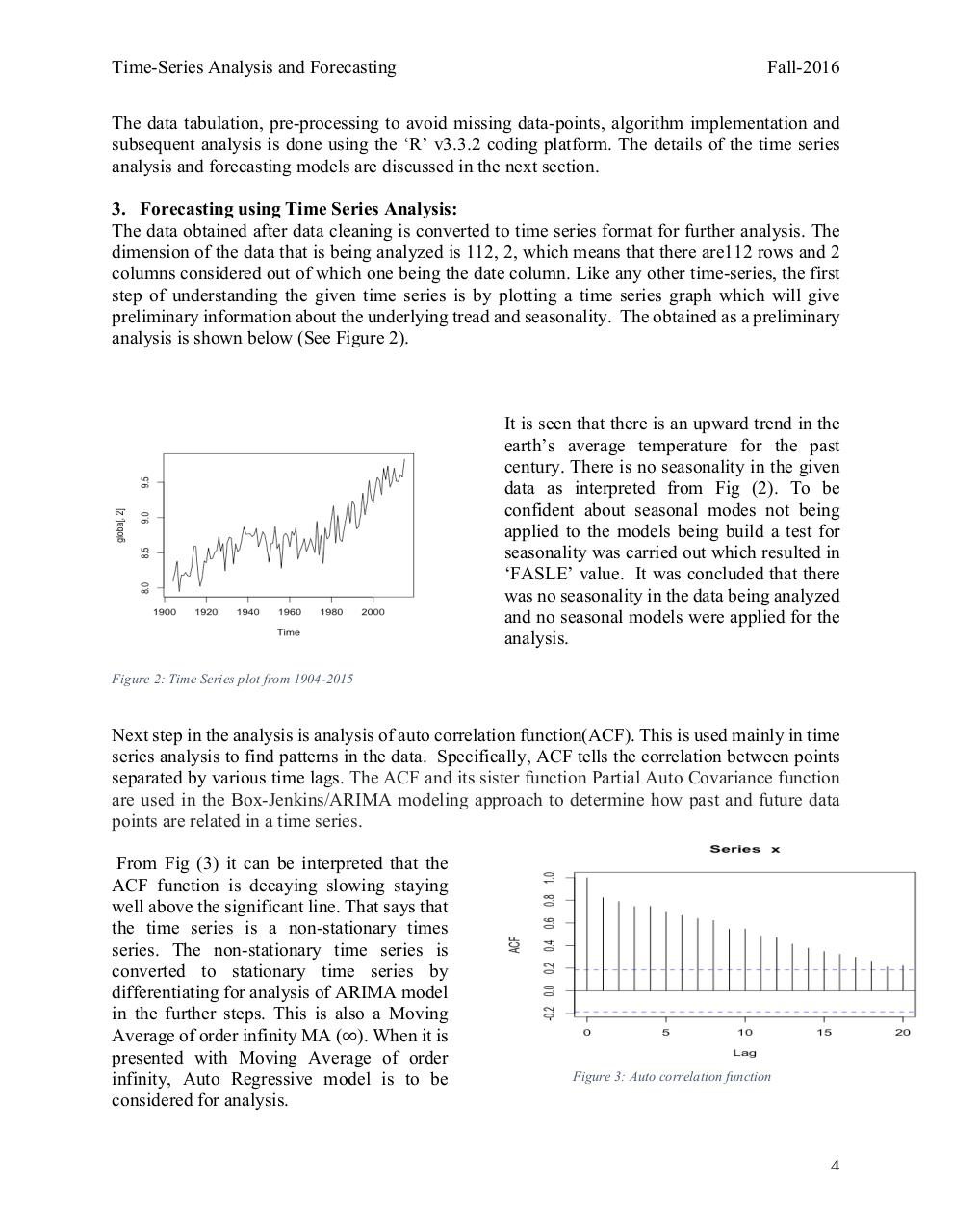# Final Report.pdfPage 1 2 3 4 5 6 7 8 9 10 11 12 13

#### Text preview

Time-Series Analysis and Forecasting

Fall-2016

The data tabulation, pre-processing to avoid missing data-points, algorithm implementation and
subsequent analysis is done using the ‘R’ v3.3.2 coding platform. The details of the time series
analysis and forecasting models are discussed in the next section.
3. Forecasting using Time Series Analysis:
The data obtained after data cleaning is converted to time series format for further analysis. The
dimension of the data that is being analyzed is 112, 2, which means that there are112 rows and 2
columns considered out of which one being the date column. Like any other time-series, the first
step of understanding the given time series is by plotting a time series graph which will give
preliminary information about the underlying tread and seasonality. The obtained as a preliminary
analysis is shown below (See Figure 2).

It is seen that there is an upward trend in the
earth’s average temperature for the past
century. There is no seasonality in the given
data as interpreted from Fig (2). To be
confident about seasonal modes not being
applied to the models being build a test for
seasonality was carried out which resulted in
‘FASLE’ value. It was concluded that there
was no seasonality in the data being analyzed
and no seasonal models were applied for the
analysis.
Figure 2: Time Series plot from 1904-2015

Next step in the analysis is analysis of auto correlation function(ACF). This is used mainly in time
series analysis to find patterns in the data. Specifically, ACF tells the correlation between points
separated by various time lags. The ACF and its sister function Partial Auto Covariance function
are used in the Box-Jenkins/ARIMA modeling approach to determine how past and future data
points are related in a time series.
From Fig (3) it can be interpreted that the
ACF function is decaying slowing staying
well above the significant line. That says that
the time series is a non-stationary times
series. The non-stationary time series is
converted to stationary time series by
differentiating for analysis of ARIMA model
in the further steps. This is also a Moving
Average of order infinity MA (∞). When it is
presented with Moving Average of order
infinity, Auto Regressive model is to be
considered for analysis.

Figure 3: Auto correlation function

4